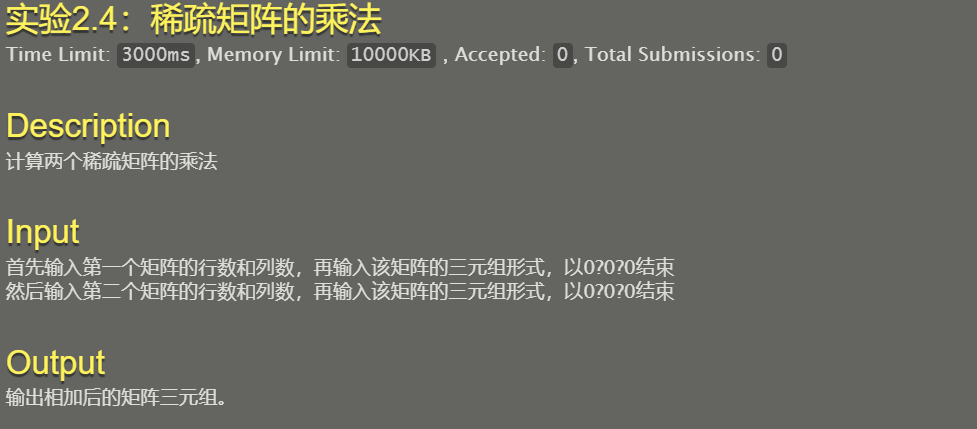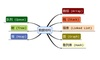• [数据结构]稀疏矩阵乘法算法实现
千次阅读
2018-06-21 20:19:56

 作者 zhonglihao 算法名 稀疏矩阵乘法 Sparse Matrix Multiplication 分类 数据结构 复杂度 O(n^2) 形式与数据结构 C++代码 一维结构体存储 特性 极简封装 不使用链表 不需要转置 计算过程容易理解 具体参考出处 《算法导论》(写的不想看) 备注

// ConsoleApplication1.cpp : 定义控制台应用程序的入口点。
//

#include "stdafx.h"
#include "stdio.h"
#include "stdlib.h"

//稀疏矩阵存储结构体 第一个元素为矩阵头，包含行列长度，元素总个数
typedef struct
{
int row;
int col;
int element;
}sparse_mat;

void SparseMatrixRectPrint(sparse_mat* s_mat);
void SparseMatrixTriPrint(sparse_mat* s_mat);
sparse_mat* SparseMatrixMul(sparse_mat* s_mat_A, sparse_mat* s_mat_B);

int _tmain(int argc, _TCHAR* argv[])
{
int i, j, k;
const int mat_A_row = 4;
const int mat_A_col = 4;
const int mat_B_row = 4;
const int mat_B_col = 4;

//原矩阵
int mat_A[mat_A_row][mat_A_col] = { 1, 1, 0, 0,
0, 0, 1, 0,
0, 1, 0, 0,
0, 0, 1, 0 };

int mat_B[mat_B_row][mat_B_col] = { 1, 0, 0, 0,
0, 1, 0, 0,
0, 0, 1, 0,
0, 0, 0, 1 };

//计算有效元素数量
int mat_A_ele_count = 0;
int mat_B_ele_count = 0;

for (i = 0; i < mat_A_row; i++)
{
for (j = 0; j < mat_A_col; j++)
{
if (mat_A[i][j] != 0) mat_A_ele_count++;
}
}

for (i = 0; i < mat_B_row; i++)
{
for (j = 0; j < mat_B_col; j++)
{
if (mat_B[i][j] != 0) mat_B_ele_count++;
}
}

//动态分配
sparse_mat* sparse_m_A = (sparse_mat*)malloc((mat_A_ele_count + 1)*sizeof(sparse_mat));
sparse_mat* sparse_m_B = (sparse_mat*)malloc((mat_B_ele_count + 1)*sizeof(sparse_mat));

//存入稀疏矩阵信息
sparse_m_A.row		= mat_A_row;
sparse_m_A.col		= mat_A_col;
sparse_m_A.element	= mat_A_ele_count;
sparse_m_B.row		= mat_B_row;
sparse_m_B.col		= mat_B_col;
sparse_m_B.element	= mat_B_ele_count;

for (i = 0, mat_A_ele_count = 0; i < mat_A_row; i++)
{
for (j = 0; j < mat_A_col; j++)
{
if (mat_A[i][j] != 0)
{
mat_A_ele_count++;
sparse_m_A[mat_A_ele_count].element = mat_A[i][j];
sparse_m_A[mat_A_ele_count].row = i;
sparse_m_A[mat_A_ele_count].col = j;
}
}
}

for (i = 0, mat_B_ele_count = 0; i < mat_B_row; i++)
{
for (j = 0; j < mat_B_col; j++)
{
if (mat_B[i][j] != 0)
{
mat_B_ele_count++;
sparse_m_B[mat_B_ele_count].element = mat_B[i][j];
sparse_m_B[mat_B_ele_count].row = i;
sparse_m_B[mat_B_ele_count].col = j;
}
}
}

//打印原数组
SparseMatrixRectPrint(sparse_m_A);
SparseMatrixRectPrint(sparse_m_B);
//SparseMatrixTriPrint(sparse_m_A);
//SparseMatrixTriPrint(sparse_m_B);

//计算稀疏矩阵乘法
sparse_mat* sparse_m_C = (sparse_mat*)SparseMatrixMul(sparse_m_A, sparse_m_B);
SparseMatrixRectPrint(sparse_m_C);

system("Pause");
return 0;
}

//三元组稀疏矩阵乘法函数 极简封装 需要花费一点时间计算申请的内存 但是肯定比链表省空间啦
//Method Written By Zhonglihao
sparse_mat* SparseMatrixMul(sparse_mat* s_mat_A, sparse_mat* s_mat_B)
{
int i, j, k;
int s_mat_C_row			= s_mat_A.row;
int s_mat_C_col			= s_mat_B.col;
int s_mat_A_ele_count	= s_mat_A.element;
int s_mat_B_ele_count   = s_mat_B.element;

//判断是否能够相乘 或 有一个全为0 那就不用乘啦
if (s_mat_A.col != s_mat_B.row) return NULL;
if (s_mat_A_ele_count == 0 || s_mat_B_ele_count == 0)
{
sparse_mat* s_mat_C	= (sparse_mat*)malloc((1)*sizeof(sparse_mat));
s_mat_C.row		= s_mat_C_row;
s_mat_C.col		= s_mat_C_col;
s_mat_C.element	= 0;
return s_mat_C;
}

//申请一个长度为B列宽的缓存 两个用途 计算输出大小时做列封禁，计算相乘时做和缓存
int* col_buffer = (int*)malloc(s_mat_C_col*sizeof(int));
//清空缓存区
for (k = 0; k < s_mat_C_col; k++) col_buffer[k] = 0;

//判断需要输出的三元大小申请内存
int malloc_element_count = 0;
for (i = 1; i <= s_mat_A_ele_count; i++)
{
if (i >= 2 && s_mat_A[i].row != s_mat_A[i - 1].row) //换行解禁
{
for (k = 0; k < s_mat_C_col; k++) col_buffer[k] = 0;
}

for (j = 1; j <= s_mat_B_ele_count; j++)
{
if ((s_mat_A[i].col == s_mat_B[j].row) && col_buffer[s_mat_B[j].col] != 1)//没有列封禁
{
col_buffer[s_mat_B[j].col] = 1;//列封禁
malloc_element_count++;
}
}
}

sparse_mat* s_mat_C		= (sparse_mat*)malloc((malloc_element_count + 1)*sizeof(sparse_mat));
s_mat_C.row			= s_mat_C_row;
s_mat_C.col			= s_mat_C_col;
s_mat_C.element		= malloc_element_count;
int s_mat_C_ele_count	= 0;//用于存入元素时做指针

//开始进行乘法相乘
for (k = 0; k < s_mat_C_col; k++) col_buffer[k] = 0;//清理列缓存
for (i = 1; i <= s_mat_A_ele_count; i++)
{
for (j = 1; j <= s_mat_B_ele_count; j++)
{
if (s_mat_A[i].col == s_mat_B[j].row)//有效用 压入缓存区
col_buffer[s_mat_B[j].col] += s_mat_A[i].element * s_mat_B[j].element;
}

//如果要换行或者是最后一行
if (((i != s_mat_A_ele_count) && (s_mat_A[i].row != s_mat_A[i + 1].row)) || i == s_mat_A_ele_count)
{
//扫描缓存组
for (k = 0; k < s_mat_C_col; k++)
{
//如果该点不是0 压入三元组 清零缓存
if (col_buffer[k] != 0)
{
s_mat_C_ele_count++;
s_mat_C[s_mat_C_ele_count].row = s_mat_A[i].row;
s_mat_C[s_mat_C_ele_count].col = k;
s_mat_C[s_mat_C_ele_count].element = col_buffer[k];
col_buffer[k] = 0;
}
}
}
}

//释放缓存 返回结果
free(col_buffer);
return s_mat_C;
}

//稀疏矩阵打印 按矩形打印 需要确定三元组按Z排列有序
void SparseMatrixRectPrint(sparse_mat* s_mat)
{
//获取行列信息
int i, j;
int row = s_mat.row;
int col = s_mat.col;

//打印元素递增 前提是三元组按照行列顺序排好，就只需要递增下标
int ele_count = 1;

//按矩阵扫描打印
for (i = 0; i < row; i++)
{
for (j = 0; j < col; j++)
{
if (i == s_mat[ele_count].row && j == s_mat[ele_count].col)
{
printf("%d\t", s_mat[ele_count].element);
ele_count++;
}
else
{
printf("0\t");
}
}//for
printf("\n");
}//for

//跳空换行 返回
printf("\n");
return;
}

//稀疏矩阵打印 按三元组结构打印
void SparseMatrixTriPrint(sparse_mat* s_mat)
{
int i, j;
int ele_count = s_mat.element;

//按顺序打印
for (i = 1; i <= ele_count; i++)
{
printf("%d\t%d\t%d\n", s_mat[i].row, s_mat[i].col, s_mat[i].element);
}

//跳空换行 返回
printf("\n");
return;
}


更多相关内容
• 某福建大三本的某三本学院的数据结构作业，题号对应清华殷人昆版。有一些可能参考借鉴了网上的代码，大体应该都能运行（尤其是大作业），仅供参考
• 数据结构稀疏矩阵乘法 1.传统矩阵相乘的算法使用三个嵌套循环实现，算法复杂度为O（m * n1 * n2） 2.使用三元组顺序表存储稀疏矩阵时，实现 Q= M * N，对于M中M（i，j）元素来说，只需要与N中第j行元素N（j,q）...

## 【数据结构】稀疏矩阵乘法

1.传统矩阵相乘的算法使用三个嵌套循环实现，算法复杂度为O（m * n1 * n2）
2.使用三元组顺序表存储稀疏矩阵时，实现 Q= M * N，对于M中M（i，j）元素来说，只需要与N中第j行元素N（j,q）相乘，再存入Q（i，q）中。为了实现这一操作，增加一个向量rpos，表示每一行的第一个非零元在三元组中的位置，rpos作用相当于快速转置中的cpot向量。
这种结构叫做 行链接的顺序表

typedef struct {
Triple data[MAXSIZE + 1]; //非零三元组表，data0未用
int rpos[MAXRC + 1];	  //各行第一个非零元的位置表
int mu, nu, tu;			  //三元组行数，列数，非零元素值
}RLSMatrix;


在创建矩阵时，需要求得矩阵的rpos向量，求法与cpot一致。

Status CreateRLSMatrix(RLSMatrix &M) {
cout << "输入矩阵行数，列数，非零元素个数：" << endl;
cin >> M.mu >> M.nu >> M.tu;
cout << "依次输入" << M.tu << "个元素的行数，列数，元素值:" << endl;
for (int i = 1; i <= M.tu; i++)
{
cin >> M.data[i].i >> M.data[i].j >> M.data[i].e;
}
int* num = (int*)malloc(M.mu * sizeof(int));
int arow;
int q;
if (M.tu) {
for ( arow = 1; arow <= M.mu; ++arow) num[arow] = 0;
for (int t = 1; t <= M.tu; ++t) ++num[M.data[t].i];//求M中每一行含非零元个数
M.rpos = 1;
//求第col列中第一个非零元在b.data 中的序号
for (arow = 2; arow <= M.mu; ++arow)M.rpos[arow] = M.rpos[arow - 1] + num[arow - 1];
}
cout << "矩阵构造完成" << endl;
return OK;
}


稀疏矩阵乘法
算法思路：
大循环是对M中的每一行进行。
rpos向量用来确定每行元素个数
再对该行每个元素M（i，j）进行处理，找到N中对应的第 j 行，将这一行所有元素与M（i，j）相乘，结果存入ctemp累加器中，该行元素都处理完成后，ctemp中所存储的便是Q中第 i 行数据

Status MultSMatrix(RLSMatrix M, RLSMatrix N, RLSMatrix &Q) {
//求稀疏矩阵乘积 Q = M x N  采用行逻辑链接存储表示
if (M.nu != N.mu)return ERROR;
Q.mu = M.mu; Q.nu = N.nu; Q.tu = 0;
int arow,tp,p,brow,t,q,ccol;
int* ctemp = (int*)malloc((M.mu+1) * sizeof(int));
if (M.tu * N.tu != 0) {
for (arow = 1; arow <= M.mu; ++arow) {
//对M的每行进行处理
for (int i = 0; i <= M.mu; i++)ctemp[i] = 0;//累加器清零
Q.rpos[arow] = Q.tu + 1;
if (arow < M.mu)tp = M.rpos[arow + 1];
else tp = M.tu + 1;//确定M中该行元素个数
for ( p = M.rpos[arow]; p < tp; p++)
{//M中对该行的每个元素进行处理
brow = M.data[p].j;
if (brow < N.mu) t = N.rpos[brow + 1];
else t = N.tu + 1;
for (q = N.rpos[brow]; q < t; ++q) {
//将该元素与矩阵N中对应行的元素进行相乘，结果存入累加器
ccol = N.data[q].j;
ctemp[ccol] += M.data[p].e *N.data[q].e;
}
}
for(ccol =1;ccol<=Q.nu;++ccol)
if (ctemp[ccol]) {
//第arow行处理完毕，将累加器中值存入Q中
if (++Q.tu > MAXSIZE)return ERROR;
Q.data[Q.tu] = { arow, ccol, ctemp[ccol] };
}//if
}//for arow
}//if
}


该算法总的时间复杂度为O（M.mu * N.nu + M.tu * N.tu/N.mu）
ctemp初始化 O（M.mu * N.nu
求Q中所有非零元 O（M.tu * N.tu / N.mu
压缩存储 O（M.mu * N.nu

展开全文• 稀疏矩阵相乘运算 一、目的 1.了解稀疏矩阵相乘运算特点 2.知道稀疏矩阵存储，创建，显示，转置方法 二、设计要求 1.问题描述 利用稀疏的特点进行存储和计算可大大节省存储空间，并实现稀疏矩阵相乘运算 2.需求分析 ...

# 一、目的

1.了解稀疏矩阵相乘运算特点
2.知道稀疏矩阵存储，创建，显示，转置方法

# 二、设计要求

## 1.问题描述

利用稀疏的特点进行存储和计算可大大节省存储空间，并实现稀疏矩阵相乘运算

## 2.需求分析

（1）用三元组顺序表表示稀疏矩阵，用来实现两个矩阵相乘运算
（2）使用三元组表示稀疏矩阵的输入形式，以阵列形式列出运算结果
（3）先让用户输入矩阵的列数和行数，给出的两个矩阵行，列数是否和所要求的相对应
（4）列出菜单项，用户根据菜单进行所有操作

# 三、概要设计

## 1.主界面设计

(1)创建矩阵，输入非零元的行数列数，以及非零元个数
(2)按照行的顺序，写出两个矩阵所在非零元的数值
(3)根据菜单，再次输入

## 2.存储结构设计

稀疏矩阵存储结构用三元组顺序表示

//三元组的定义：
typedef  struct
{
int  row; //行下标
int  col; //列下标
int  e; //元素值
}Triple;
//矩阵的定义;
typedef  struct
{
Triple  data[MAXSIZE]; //三元组表
int m ,n ,len; //行,列,非零元个数
}TSMatrix;


## 3.系统功能设计

此系统通过菜单让用户输入数据后创建两个矩阵，接着对两个矩阵相乘运算，并输出结果，以此
实现以下功能
（1）首先创建两个矩阵，用户输入的行和列数，非0元个数，以及所有非零元的行列值
（2）其次两个矩阵相乘，由mult函数实现，输出矩阵A,B及（A*B）的阵列形式

# 四、模块设计

## 1.模块设计

此程序包含两个模块，主程序模块，矩阵运算模块
其调用关系是：主程序模块→矩阵运算模块

## 2.系统子程序及功能设计

此系统一共有7个子程序，子程序的功能和函数名如下：
（1）矩阵初始化
void initMatrix(TSMatrix *A)
（2）创建矩阵，调用(1)
void createTSMatrix(TSMatrix *A)
（3）找到m行n列元素在矩阵A的三元组表中的位置
int search(TSMatrix *A,int m, int n)
（4）两个矩阵相乘，调用(3)
void mult(TSMatrix *A,TSMatrix *B,TSMatrix *C)
（5）输出的矩阵
void print(TSMatrix *A)
（6）显示程序主函数，工作区函数
void showtip()
（7）主函数
void main()

## 3.函数主要调用关系

主函数7调用函数2，4，5，6
函数2在调用函数1，函数4再调用函数3

# 五、详细设计

## 1.数据类型定义

采用矩阵的三元组顺序表存储结构

//三元组的定义：
typedef  struct
{
int  row; //行下标
int  col; //列下标
int  e; //元素值
}Triple;
//矩阵的定义;
typedef  struct
{
Triple  data[MAXSIZE]; //三元组表
int m ,n ,len; //行,列,非零元个数
}TSMatrix;


## 2.主要子程序详细设计

（1）主函数模块设计

int main()
{
TSMatrix A,B,C;
createTSMatrix(&A);
createTSMatrix(&B);
MulTSMatrix(&A,&B,&C);
printMatrix(&C);
return 0;
}


（2）创建矩阵

void createTSMatrix(TSMatrix *A)//创建矩阵
{    int i=0;     //data未用
scanf("%d?%d",&A->m,&A->n);
int flag = 1;
int a,b,c;
char c1,c2,c3;
while(flag)
{
scanf("%d?%d?%d",&a,&b,&c);
if(a==0&&b==0&&c==0)
break;
i++;
A->data[i].row=a;
A->data[i].col=b;
A->data[i].e=c;
}
A->len=i;
}


（3）输出矩阵

void print(TSMatrix *B)//输出矩阵
{
for(int i=1;i<=B->len;i++)
{
printf("%d?%d?%d\n",B->data[i].row,B->data[i].col,B->data[i].e);
}
}


(4)矩阵相乘

void mult(TSMatrix *A,TSMatrix *B,TSMatrix *C)//矩阵相乘
{
C->len=0;
int p,q,x,cnt;
int i1,j1;
int sum;
cnt=1;
for(int i=1;i<=A->m;i++)
{
for(int j=1;j<=B->n;j++)//遍历每行每列
{
sum=0;
for(int k=1;k<=A->n;k++)//每个行与每个列单个数的遍历
{    p=0;q=0;
for(i1=1;i1<=A->len;i1++){  //遍历A找符合i行k列的数
if(A->data[i1].row==i&&A->data[i1].col==k) p=A->data[i1].e;
}
for(j1=1;j1<=B->len;j1++){//遍历B找符合k行j列的数
if(B->data[j1].row==k&&B->data[j1].col==j) q=B->data[j1].e;
}
sum=sum+(p*q);
}
if(sum!=0) {cnt++;
C->data[cnt].e=sum;
C->data[cnt].row=i;
C->data[cnt].col=j;
}
}
C->len=cnt;
}


展开全文• C语言数据结构之两个稀疏矩阵相加。代码中代码功能描述、输入输出说明和测试输出输入。
• 稀疏矩阵相乘包含1.目的 2.设计要求 3.概要设计 4.模块设计 5.详细设计 6.测试分析 7.设计总结
• //稀疏矩阵乘法（三元组） #include <stdio.h> #include <stdlib.h> #define max 200 typedef struct Triple{ int i,j;//i行j列 int e; }Triple; typedef struct TSMaTrix{ Triple elem[max]; int ...//稀疏矩阵乘法（三元组）
#include <stdio.h>
#include <stdlib.h>

#define max 200
typedef struct Triple{
int i,j;//i行j列
int e;
}Triple;
typedef struct TSMaTrix{
Triple elem[max];
int rpos[max]; //行逻辑链接的顺序表,保存每行起始地址
int mu,nu,tu;
}TSMatrix,*TSMatrixPtr;

TSMatrixPtr Matrix_Init(TSMatrixPtr M){
M = (TSMatrixPtr)malloc(sizeof(TSMatrix));
M->mu = 0;
M->nu = 0;
M->tu = 0;
int i,j,x;
scanf("%d %d",&M->mu,&M->nu);
while(1){
scanf("%d %d %d",&i,&j,&x);
if(i == 0&&j==0&&x==0)
break;
else
{
M->elem[M->tu].i = i;
M->elem[M->tu].j = j;
M->elem[M->tu].e = x;
M->tu++;
}
}
//计算rpos；
int num;								//num[]保存每一行的非零元素个数  cpot[]保存每行元素起始地址
for (int i = 0; i < M->mu; i++)
num[i] = 0;								    //对num初始化
for (int j = 0; j < M->tu; j++)
num[M->elem[j].i]++;
M->rpos = 0;
for (int k = 1; k <= M->mu; k++)
M->rpos[k] = M->rpos[k - 1] + num[k - 1];

return M;
}

TSMatrixPtr MultiMatrix(TSMatrixPtr A,TSMatrixPtr B,TSMatrixPtr C){
C = (TSMatrixPtr)malloc(sizeof(TSMatrix));
if(A->nu != B->mu)
printf("error");
float temp[max];
for(int i = 0;i<max;i++)//初始化
temp[i] = 0;

C->mu = A->mu;
C->nu = B->nu;
C->tu = 0;
C->rpos = 0;

int arow,brow,ccol,p,q,ta,tb;

if(A->tu*B->tu != 0){
for(arow=1;arow <= A->mu;arow++)
{
for(int i = 0;i<max;i++)//初始化
temp[i] = 0;
C->rpos[arow] = C->tu;

if (arow < A->mu)						//获得A的该行非0元数
ta = A->rpos[arow + 1];
else
ta = A->tu;
for(p = A->rpos[arow] ; p < ta ; ++p)//A->elem[p];
{
brow = A->elem[p].j;
if (brow < B->mu)									//取B的列标行的非0数
tb = B->rpos[brow + 1];
else
tb = B->tu;
for(q = B->rpos[brow];q < tb ;++q)
{
ccol = B->elem[q].j;
temp[ccol] +=A->elem[p].e * B->elem[q].e;//累加
}

}

for(ccol = 1;ccol <= C->nu; ccol++)
{
if(temp[ccol] != 0)
{
C->elem[C->tu].i = arow;
C->elem[C->tu].j = ccol;
C->elem[C->tu].e = temp[ccol];
C->tu++;
}
}
}
}
return C;
}

void Print_List(TSMatrixPtr M){
for(int i = 0;i<M->tu;i++)
{
printf("%d %d %d\n",M->elem[i].i,M->elem[i].j,M->elem[i].e);
}
}
int main(){
TSMatrixPtr A,B,C;
A = Matrix_Init(A);
B = Matrix_Init(B);
C = MultiMatrix(A,B,C);
Print_List(C);

return 0;
}

展开全文• = N.mu)//矩阵M的列数与矩阵N的行数不等，则不能做矩阵乘运算 return ERROR; Q.mu = M.mu; Q.nu = N.nu; Q.tu = 0; if(M.tu * N.tu == 0)//其中任意矩阵的元素个数为零，则不能做乘运算 return ERROR; else ...
• 清华大学出版社数据结构稀疏矩阵相乘编程，程序不大。。。
• 实验2.4：稀疏矩阵的乘法Time Limit: 3000ms, Memory Limit: 10000KB , Accepted: 0, Total Submissions: 0Description计算两个稀疏矩阵的乘法Input首先输入第一个矩阵的行数和列数，再输入该矩阵的三元组形式，...
• 但上面的算法内层循环里遍历每一行来检查每一行是否在对应的列中有元素，对于稀疏矩阵（大部分元素为nil或0的矩阵），这个循环除了遍历少量的非0元素，还遍历了很多的0元素，所以做简单的调换，避免遍历列： ...lua
• 数据结构稀疏矩阵基本运算实验报告.课 程 设 计课程：数据结构题目：稀疏矩阵4 三元组单链表 结构体(行数、列数、头) 矩阵运算重载运算符 优班级：姓名：学号：设计时间：2010年1月17日——2010年5月XX日成绩：指导...
• 本实验要求实现两个稀疏矩阵相乘积的算法。其中稀疏矩阵非零元素数量小于100. 输入： 第1个稀疏矩阵的行数 列数 非零元个数(三个数都大于0) 三元组 第2个稀疏矩阵的行数 列数 非零元个数(三...
• 稀疏矩阵的加法和乘法运算 上节学了类的友元，这节开始矩阵加法和乘法运算： （一）加法： 在这里插入代码片 在这里插入代码片算法
• [NOJ]数据结构实验2.4 稀疏矩阵的乘法 #include<stdio.h> #include<stdlib.h> typedef struct OLNode { int row,col; int value; struct OLNode *right,*down; }OLNode,*OLink;...
• 数据结构试验中稀疏矩阵相乘的程序清单，多多学习，多多进步
• 数据结构实验报告 稀疏矩阵计算器。稀疏矩阵是指那些多数元素为零的矩阵。利用“稀疏”特点进行存储（只存储非零元）和计算可以大大节省存储空间，提高计算效率。实现一个能进行稀疏矩阵基本运算的运算器。以“带行...
• 数据结构课程设计说明书 题 目稀疏矩阵运算器设计 学生姓名 学 号 专 业计算机科学与技术 班 级计 09-1 班 指导教师刘 月 峰 2011 年 6 月 24 日 稀疏矩阵运算器设计 摘 要 摘要设计一稀疏矩阵运算器实现转置相加...
• 说明如果在矩阵中，多数的元素并没有资料，称此矩阵为稀疏矩阵(sparse matrix)， 由于矩阵在程式中常使用二维阵列表示，二维阵列的大小与使用的记忆体空间成正比，如果多数的元素没有资料，则会造成记忆体空间的浪费...
• 按照教科书《数据结构(C语言版)》(严蔚敏等)5.3.2节中描述的方法，以十字链表表示稀疏矩阵。一、需求分析稀疏矩阵是指那些多数元素为零的矩阵。利用“稀疏”特点进修学校储存和计算可以大大节省储存空间，提高计算...
• 参考《数据结构(C语言版)》- 严蔚敏 吴伟民 - 清华大学出版社 稀疏矩阵的结构定义 typedef struct { int i, j; //该非零元的行下标和列下标 ElemType e; //非零元对应的值 }Triple; typedef struct { Triple ...
• ## 稀疏矩阵相乘

千次阅读 2017-11-14 18:59:57
对于用三元组实现稀疏矩阵相乘的算法，首先构造一个结构体，结构图体中应该有五个量。其中第一个量是表示稀疏矩阵常用的三元组,定义如下：typedef struct{ int i, j; //该非零元素行列下标 ElemType e; }Triple; /...算法 c++
• 请编写并测试一个稀疏矩阵相乘的函数 matrix sparse_matrix_mul(const matrix&m1, constmatrix& m2) 其中matrix为一个描述稀疏矩阵的结构体： struct matrix { float* _elements; //矩阵中所有元素的数组（按列...测试 函数 matrix
• 题目如下： 看一下题目，就是三元组表稀疏矩阵的乘法，然后输出。 首先将矩阵每行的非0元个数记下来，那么矩阵每行第一个非0元在三元表的位置就是上一行非0元个数加上上一行首个非0元位置之和。这样就可以不用遍历...
• 题目：假设稀疏矩阵A和B均以三元组表作为存储结构，试写出矩阵相加和相乘的算法，另设三元组表C存放结果矩阵。要求：从键盘输入稀疏矩阵A和B检测A和B能否相加/相乘如能，做矩阵相加和相乘运算，并打印运算结果如不能...
•c++
• 数据结构课程设计说明书 题 目稀疏矩阵运算器设计 学生姓名 学 号 专 业计算机科学与技术 班 级计 09-1 班 指导教师刘 月 峰 2011 年 6 月 24 日 稀疏矩阵运算器设计 摘 要 摘要设计一稀疏矩阵运算器实现转置相加...
• 完成了加减乘，绝对无误 1、 加法：可以完成加法的情况下分别对每行处理，如果非零员的列标小则在插入之，相同则完成加法运算，如果结果非零则插入。否则继续下一个飞灵员的...最后用稀疏矩阵的存储方法保存非零员。...

# 数据结构稀疏矩阵相乘数据结构 订阅